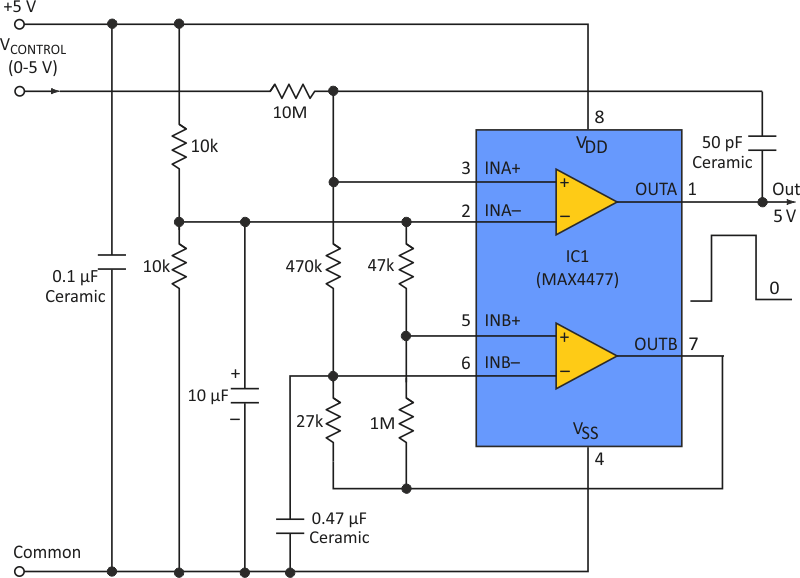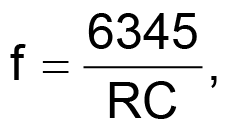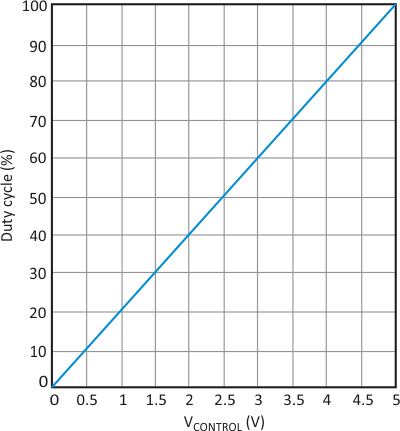# Simple PWM Modulator Allows DC Control Signal To Drive LEDs

## Maxim MAX4477

LED-driver circuits can be dimmed by applying a variable duty cycle (pulse-width modulation, or PWM) to the LED. PWM exploits LED behavior: At higher current levels, the LED’s light output is higher for a given level of power dissipation (temperature). Thus, applying PWM current to the LED yields an average power comparable to that of dc control, but with higher operating current and greater light output.

Even if the available control signal is a dc level, you can implement PWM control with a simple circuit that provides predictable behavior and good linearity. Consisting of a dual comparator and a few external components, the circuit produces a 500-Hz PWM signal with 2% nonlinearity and a duty cycle adjustable from 0% to 100%, using a 0- to 5-V control signal (Fig. 1).Figure 1. This circuit converts a 0- to 5-V dc control signal to a 500-Hz PWM signal that is able to drive LEDs.

The comparator’s “B” side is configured as an oscillator running at about 500 Hz. It produces a triangular waveform at the positive input, with an amplitude about one-tenth the supply voltage. The “A” side compares the triangle waveform with the dc control signal and generates a PWM signal at OUTA. The PWM generator and dc control-signal generator should run off the same +5-V supply.

You can adjust the oscillation frequency (f) by changing the value of capacitor C in the equation:where R is in kilohms, C is in microfarads, and f is in kilohertz. To get 500 Hz in the example circuit, C = 0.47 µF and R = 27 kΩ.Figure 2. A graph of duty cycle versus control voltage shows that the circuit’s linearity is about 2%.

Figure 2 shows the circuit response as PWM duty cycle versus dc control-signal level.

## Materials on the topic

Electronic Design